# Canvas Arc in HTML5

### Canvas Arc

It is used to create the part of the circle or half of the circle

Arc uses the 2*Math.PI and Math.PI and the arc uses the stroke and fill methods to create the arc.

```<canvas id="myCanvas" width="400" height="250" style="border:5px solid blue;">
</canvas>
<script>
var canvas = document.getElementById("myCanvas");
var context = canvas.getContext("2d");

context.beginPath();
context.arc(100, 75, 50, 0, 2 * Math.PI);
context.stroke();
</script>
```
• In the above code snippet we have defined the canvas arc with the canvas id  as `"mycanvas"` with the width as `400` and height as` 250` with the border as 5px solid blue
• To create the arc we have the folloing code as
• `context.arc(100, 75, 50, 0, 2 * Math.PI);` - It defines the arc with the given X values
• `2*Math.PI` defines the circle and `Math.PI` defines the semi circle

output### X - coordinate of the arc

The x- coordinate of the arc defines moving the arc from left side of the canvas by increasing the values of the x

``` <h2 style="color:green">x-coordinate moves right from the canvas  </h2>
<canvas id="myCanvas" width="400" height="250" style="border:5px solid red;">
</canvas>
<script>
var canvas = document.getElementById('myCanvas');
var context = canvas.getContext('2d');
context.beginPath();
context.arc(300, 75, 50, 0 * Math.PI, 1.5 * Math.PI);
context.stroke();
</script>
```

X- Coordinate

• In the above code snippet we have defined the canvas arc with` x coordinate` moving from left side of the canvas to right side
• `context.arc(300, 75, 50, 0 * Math.PI, 1.5 * Math.PI);` - Context(x, y, r,Math.PI, 2*Math.PI) the x value moves right when the x value is increased and it moves left side when the x value is decreased

output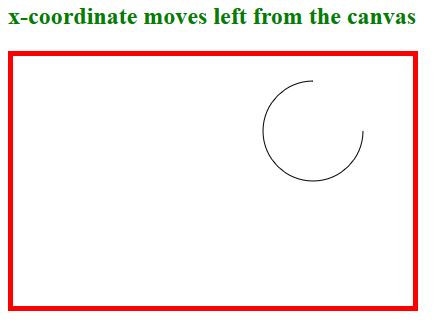### Y-Coordinate of the arc

Y- Coordinate is used to move the arc from top to bottom when we increase the value, to move the arc from bottom to top we need to decrease the value

```  <h2 style="color:green">Y-coordinate moves the arc from top to bottom in the canvas  </h2>
<canvas id="myCanvas" width="400" height="250" style="border:5px solid red;">
</canvas>
<script>
var canvas = document.getElementById('myCanvas');
var context = canvas.getContext('2d');
context.beginPath();
context.arc(100, 95, 50, 0 * Math.PI, 1.5 * Math.PI);
context.stroke();
</script>

```

Y-coordinate

• In the above code snippet we have defined the y-coordinate which moves  the arc from top to bottom when we increase the value, to move the arc from bottom to top we need to decrease the value
• `context.arc(100, 95, 50, 0 * Math.PI, 1.5 * Math.PI);` - The y value` "95"` which increments the value from top to bottom in the canvas

output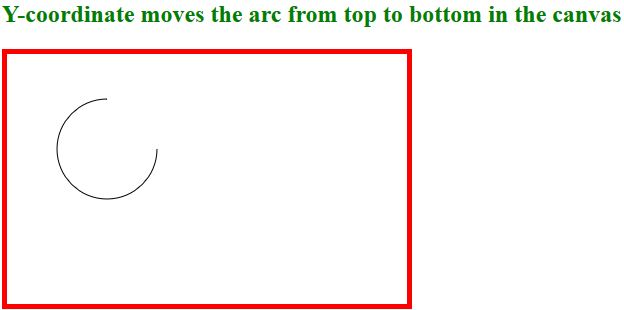To create radius we need to define the width and height of the object, the radius of the arc is a part of the circle

``` <h2 style="color:green">Radius of the arc </h2>
<canvas id="myCanvas" width="400" height="250" style="border:5px solid red;">
</canvas>
<script>
var canvas = document.getElementById('myCanvas');
var context = canvas.getContext('2d');
context.beginPath();
context.arc(100, 95, 75, 0 * Math.PI, 1.5 * Math.PI);
context.stroke();
</script>
```

• In the above code snippet we have defined the `radius` of the arc, We are having the `canvas` id as `"mycanvas"` and the width as `400` and height as `250`
• var canvas = document.getElementById('myCanvas'); - it defines the canvas as with the id as mycanvas
• var context = canvas.getContext('2d'); - it defines the context to the canvas element
• context.beginPath(); - It begins the path
• `context.arc(100, 95, 50, 0 * Math.PI, 1.5 * Math.PI);` - It defines the arc with the given values in the canvas element
• The radius value here is `"75"` which is used to define the` size` of the radius of the arc

output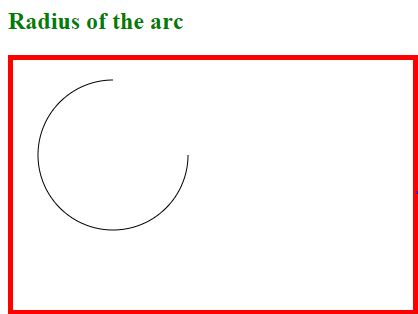sAngle

It is used as projecting an definite angle by giving the values in the arc

``` <h2 style="color:green">sAngle of the Clock is used to project an angle in a shape  </h2>
<canvas id="myCanvas" width="400" height="250" style="border:5px solid red;">
</canvas>
<script>
var canvas = document.getElementById('myCanvas');
var context = canvas.getContext('2d');
context.beginPath();
context.arc(100, 95, 75, 0.4 * Math.PI, 2 * Math.PI);
context.stroke();
</script>
```

sAngle

• In the above code snippet we have defined the sAngle of the arc, We are having the `canvas` id as `"mycanvas"` and the width as `400` and height as `250`
• `var canvas = document.getElementById('myCanvas');` - it defines the canvas as with the id as mycanvas
• `var context = canvas.getContext('2d');` - it defines the context to the canvas element
• `context.beginPath();` - It begins the path
• `context.arc(100, 95, 50, 0 * Math.PI, 1.5 * Math.PI);`- It defines the arc with the given values in the canvas element
• The `sAngle` is used to create the shape of the arc `(0*Math.PI)` is used to create the shape of the angle

output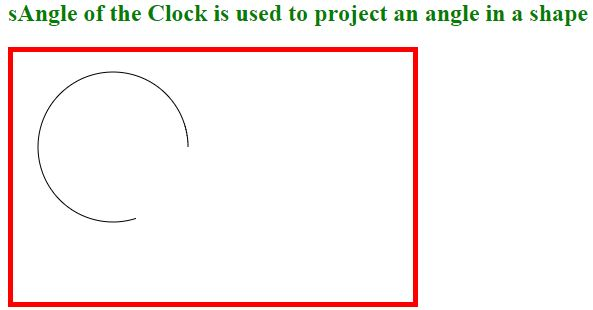### eAngle

It is used to end the circle by using the 2*Math.PI

```<h2 style="color:green">sAngle of the Clock is used to project an angle in a shape  </h2>
<canvas id="myCanvas" width="400" height="250" style="border:5px solid red;">
</canvas>
<script>
var canvas = document.getElementById('myCanvas');
var context = canvas.getContext('2d');
context.beginPath();
context.arc(100, 95, 75, 0* Math.PI, 2 * Math.PI);
context.stroke();
</script>
```

eAngle

• In the above code snippet we have defined the sAngle of the arc, We are having the `canvas` id as `"mycanvas"` and the width as `400` and height as `250`
• `var canvas = document.getElementById('myCanvas');` - it defines the canvas as with the id as mycanvas
• `var context = canvas.getContext('2d');` - it defines the context to the canvas element
• `context.beginPath();` - It begins the path
• `context.arc(100, 95, 50, 0 * Math.PI, 2 * Math.PI);`- It defines the arc with the given values in the canvas element
• The` eAngle` is used to create the shape of the arc `2*Math.PI`  is used to create the shape of the angle

output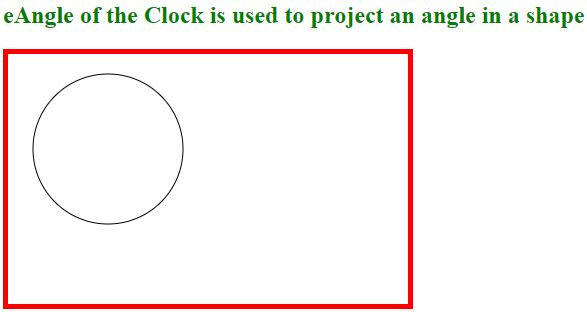### Counter clock wise or Anti clock wise

It Specifies whether the drawing should be counter clockwise or clockwise. False is default, and indicates clockwise, while true indicates counter-clockwise/anti-clock wise

```<h2 style="color:green">Counter clockwise </h2>
<canvas id="myCanvas" width="400" height="250" style="border:5px solid red;">
</canvas>
<script>
var canvas = document.getElementById('myCanvas');
var context = canvas.getContext('2d');
context.beginPath();
context.arc(100, 75, 50, 1.8 * Math.PI, 2.2 * Math.PI, true);
context.stroke();
</script>
```

Counter Clockwise

• In the above code snippet we have defined the sAngle of the arc, We are having the `canvas` id as `"mycanvas"` and the width as `400` and height as `250`
• `var canvas = document.getElementById('myCanvas');` - it defines the canvas as with the id as mycanvas
• `var context = canvas.getContext('2d');` - it defines the context to the canvas element
• `context.beginPath();` - It begins the path
• `context.arc(100, 75, 50, 1.8 * Math.PI, 2.2 * Math.PI, true)``;`- It defines the arc with the given values in the canvas element
• The` true` is used to indicate clock counter clockwise

output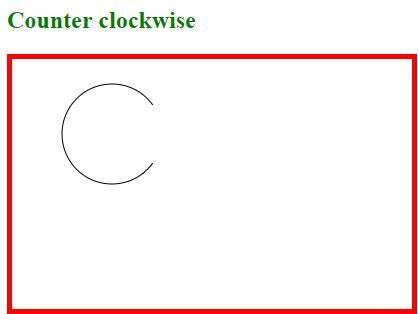Views: 2825 | Post Order: 44